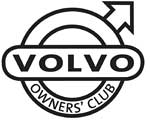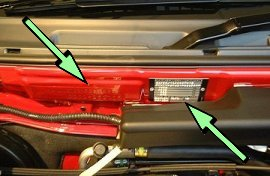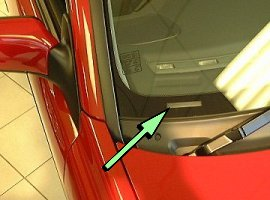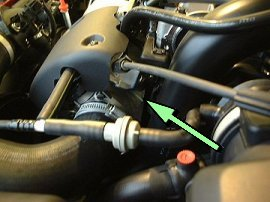# Volvo S40 V40 V50 VIN Plates

1800  |  200  |  300  |  400  |  700  |  850 - 900  |  40/50  |  60  |  70  |  80  |  90  |  XC90

This information will help you to understand the information on the VIN plate of your Volvo car.

Click on the model series number above

Example VIN Number:

YV1VS1218TF123456

YV1 V S 12 1 3 T F 123456
Manufacturer Code Vehicle Type Doors Engine Type Emission Gearbox Model Year Factory Code Chassis umber

Manufacturer Code
YV1 = cars YV2 = trucks YV3 = buses YV4 = multipurpose 4V1 = trucks 4V2 = 4V3 =
4V4 = trucks 4V5 = trucks 4V6 = 4VL = 4VM = 4VZ = MHA = PT. Central Sole Agency

Vehicle Type
V = S40/V40 M = S40 (2004 on)

Doors
S = 4DRS W = 5DRS S = S40 (2004 on) W = V50 (2004 on)

Engine Type
10 = B4164S2 11 = B4164S 12 = B4184S 13 = B4184SM 14 = B4184S2 15 = B4184S3 16 = B4204S 17 = B4204S2 18 = B4194T 19 = B4204T 24 = B4194T2
25 = B4204T2 EXC (USA)(CDN) 25 = B4204T2 CVVT (USA)(CDN) 26 = B4184SJ 27 = B4204T4 (USA)(CDN) 28 = B4184S10 29 = B4204T3 EXC (USA)(CDN) 29 = B4204T3 USA (USA)(CDN) 30 = B4184S9 (USA)(CDN) 30 = B4184S9 37 = B4204T5 38 = B5244S4
39 = B5244S7 66 = B5244S5 68 = B5254T3 70 = D4192T3 71 = D4192T 72 = D4204T2 73 = D4192T2 75 = D4204T 78 = D4192T4 --- ---

Emissions to 2004
0 = KOD: EM I1 1 = KOD: EM H1 2 = KOD: EM C1 4 = KOD: EM P1 5 = KOD: EM R1 6 = KOD: EM U1 7 = KOD: EM P2 8 = KOD: EM H2 E = KOD: EM N3 K = KOD: EM L4

Emissions from 2004
0 = KOD: SULEV+ 2 = KOD: ULEV2 3 = KOD: EURO3 4 = KOD: EURO4 5 = KOD: EURO5

Gearbox
0 = Manual gearbox 1 = M66, MMT6 manual gearbox 2 = M56 manual gearbox 3 = M3P, M5P, M5D manual gearbox 5 = M5M42 manual gearbox 6 = AW50-42 automatic gearbox 9 = AW55-50SN automatic gearbox

Model Year and Chassis Number
T = 1996. S40, V40 CH 000- V = 1997. S40, V40 CH 31000- W = 1998. S40, V40 CH 130000- X = 1999. S40, V40 CH 315000- Y = 2000. S40, V40 CH 415000- 1 = 2001. S40, V40 CH 600000- 12 = 2002. S40, V40 CH 800000- 3 = 2003. S40, V40 CH 920000- 4 = 2004/ S40, V40 CH 020000-

Model Year From 1980
A = 1980 B = 1981 C = 1982 D = 1983 E = 1984 F = 1985 G = 1986 H = 1987 J = 1988 K = 1989 L = 1990 M = 1991
N = 1992 P = 1993 R = 1994 S = 1995 T = 1996 V = 1997 W = 1998 X = 1999 Y = 2000 1 = 2001 2 = 2002 3 = 2003
4 = 2004 5 = 2005 6 = 2006 7 = 2007 8 = 2008 9 = 2009 A = 2010 (code restarted) B = 2011 C = 2012 D = 2013 E = 2014 ---

Factory Code
0 = Sweden, Kalmar Plant 1 = Sweden, Torslanda Plant VCT 21(Volvo Torslandaverken) (Gothenburg) 2 = Belgium, Ghent Plant VCG 22 3 = Canada, Hatdfax Plant 4 = Italy, - Bertone models 240 5 = Malaysia, 6 = Australia,
7 = Indonesia, A = Sweden, Uddevalla Plant (Volvo Cars/TWR (Tom Walkinshaw Racing)) B = Italy, - Bertone Chongq 31 D = Italy, - Bertone models 780 E = Singapore, F = The Netherlands, Born Plant (NEDCAR) J = Sweden, Uddevalla Plant VCU 38 (Volvo Cars/ Pininfarina Sverige AB)

Engine VIN
01 = B18K 02 = B18KP 03 = B18KPD 04 = B18ES 05 = B18ED 06 = B18FTM 07 = B18KD 10 = B18U (M)-200/203 11 = B17A 12 = B18U (M)-103/113
14 = B18E/EP 15 = B18U -200/203 16 = B18U -103/113 17 = B16F 18 = B18F/FP 19 = B18FT 20 = B200 K/O 21 = B19A 22 = B200 E/O 23 = 200 E/O
24 = B19E 25 = B200 E/A 26 = B19ET 27 = B200F 28 = B200 E/E 31 = B20F 32 = B20F (M) 37 = D20 41 = B21A 44 = B21E
45 = B21F 46 = B21ET 47 = B21FT 48 = B21LH Jet 49 = B21F MPG (1981), B21F Kjet (1982) 53 = B14.4E 54 = B14.40 55 = B14.4S 61 = B27A 62 = B28A
64 = B27E 65 = B27F 68 = B28E 69 = B28F 70 = D19T (EGR) 72 = D16 74 = D24 TIC with EGR 75 = D24 TIC without EGR 76 = D24T 77 = D24 (1981-1984)
79 = D19T 80 = B230G 81 = B23A 82 = B230GT & B230FD 83 = B230FD w/ pulsair 84 = B23E 85 = B230FB 86 = B230FT - EGR 87 = B230FT - non EGR 88 = B23F (1983-1984), B230F (1985-95)
89 = B234F 92 = B6244F 93 = B6254F 95 = B6304F with air pump 96 = B6204F without air pump 98 = N6304G 99 = B6254G --- --- ---

1996-2012

Location of Chassis/VIN Plate
On the centre of the bulkhead on a metal plate held by two silver rivets.Stamped Chassis Number
Stamped into the offside centre of the bulkhead to the left of the VIN plate.

Visible VIN
Viewed through the nearside or offside base of the front windscreen.Location of Engine Number
On the front nearside of the block.